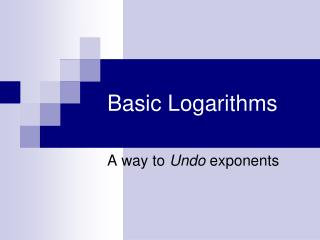Download PresentationBasic Logarithms

# Basic Logarithms

Download Presentation## Basic Logarithms

- - - - - - - - - - - - - - - - - - - - - - - - - - - E N D - - - - - - - - - - - - - - - - - - - - - - - - - - -
##### Presentation Transcript

1. Basic Logarithms A way to Undo exponents

2. Subtraction is the inverse of addition

3. When you were in grade school, you probably learned about subtraction this way. 2 + = 8 7 + = 10

4. Then one day your teacher introduced you to a new symbol ─ to undo addition

5. 3 + = 10 Could be written 10 ─ 3 =

6. 8 – 2 =

7. 8 – 2 = 2 + ? = 8

8. 8 – 2 = 2 + 6 = 8

9. 8 – 2 = 6 2 + 6 = 8

10. The same could be said about division÷

11. 40 ÷ 5 =

12. 40 ÷ 5 = 5 x ? = 40

13. 40 ÷ 5 = 5 x 8 = 40

14. 40 ÷ 5 = 8 5 x 8 = 40

15. Consider √49

16. = ?

17. = ? ?2 = 49

18. = ? 72 = 49

19. = 7 72 = 49

20. Exponential Equations: 5? = 25

21. Exponential Equations: 52 = 25

22. Logarithmic Form of 52= 25 islog525 = 2

23. log525 =?

24. log525 =? 5? = 25

25. log525 =? 52 = 25

26. log525 =2 52 = 25

27. Try this one…

28. log749 =?

29. log749 =? 7? = 49

30. log749 =? 72 = 49

31. log749 =2 72 = 49

32. and this one…

33. log327 =?

34. log327 =? 3? = 27

35. log327 =? 33 = 27

36. log327 =3 33 = 27

37. Remember your exponent rules? 70 = ? 50 = ?

38. Remember your exponent rules? 70 = 1 50 = 1

39. log71 =?

40. log71 =? 7? = 1

41. log71 =? 70 = 1

42. log71 =0 70 = 1

43. Keep going…

44. log31 =?

45. log31 =? 3? = 1

46. log31 =? 30 = 1

47. log31 =0 30 = 1

48. Remember this? 1/25 = 1/ 52=5-2

49. log5()=?